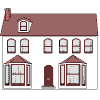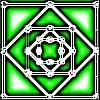#### You may also like### Homes

Six new homes are being built! They can be detached, semi-detached or terraced houses. How many different combinations of these can you find?### Number Squares

Start with four numbers at the corners of a square and put the total of two corners in the middle of that side. Keep going... Can you estimate what the size of the last four numbers will be?### I'm Eight

Find a great variety of ways of asking questions which make 8.

# Fair Exchange

##### Age 5 to 7Challenge Level

Jamie from Waddington Redwood Primary told us:

I used a 1p and two 2p coins for 5p.
I used four 2p coins for 8p.
I used five 2p coins and a 1p for 11p.

Pranav from Vardhana School looked at how many different ways you can make each total:

First, we must find the individual values of each number:
The 5 coin can be made with:

5
2+2+1
2+1+1+1
1+1+1+1+1

which makes 4 ways.

The 2 coin can be made with:

2
1+1

which makes 2 ways.

The 1 coin can be made with:

1

which makes just 1 way.

This is really helpful, Pranav. Pranav then went on to make a table of the number of ways the values from 1 up to 14 could be made with the coins. However, it's very difficult to make sure that we don't count some ways which are the same twice.

Meg used Pranav's method to find all the ways of making 8 and 11 with these coins, and wrote a list.

To make sure I count all the ways to make 8 and don't count any twice, I'll first list all the ways which use 5p, then list the ways that don't use 5p. I found that there are seven possible ways to do this. Here is the list:

5 + 2 + 1
5 + 1 + 1 + 1
2 + 2 + 2 + 2
2 + 2 + 2 + 1 + 1
2 + 2 + 1 + 1 + 1 + 1
2 + 1 + 1 + 1 + 1 + 1 + 1
1 + 1 + 1 + 1 + 1 + 1 + 1 + 1

Then I did a similar thing for 11p. First I listed the ways using two 5ps (one way only), then the ways which use one 5p, then the ways that don't use any 5ps at all. I found that there are eleven possible ways to do this in total. Here is the list:

5 + 5 + 1
5 + 2 + 2 + 2
5 + 2 + 2 + 1 + 1
5 + 2 + 1 + 1 + 1 + 1
5 + 1 + 1 + 1 + 1 + 1 + 1
2 + 2 + 2 + 2 + 2 + 1
2 + 2 + 2 + 2 + 1 + 1 + 1
2 + 2 + 2 + 1 + 1 + 1 + 1 + 1
2 + 2 + 1 + 1 + 1 + 1 + 1 + 1 + 1
2 + 1 + 1 + 1 + 1 + 1 + 1 + 1 + 1 + 1
1 + 1 + 1 + 1 + 1 + 1 + 1 + 1 + 1 + 1 + 1

Well done everyone for these solutions! If you have had a go at this puzzle, why not challenge yourself with some different totals, or even some different coins?